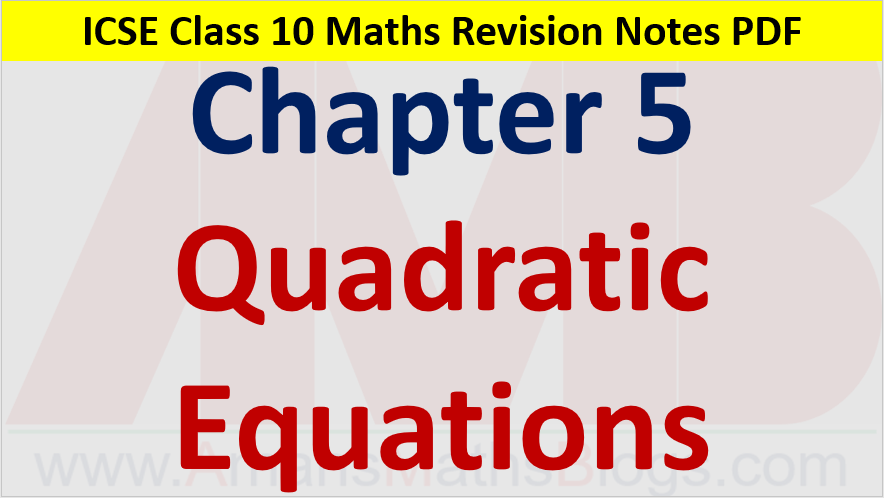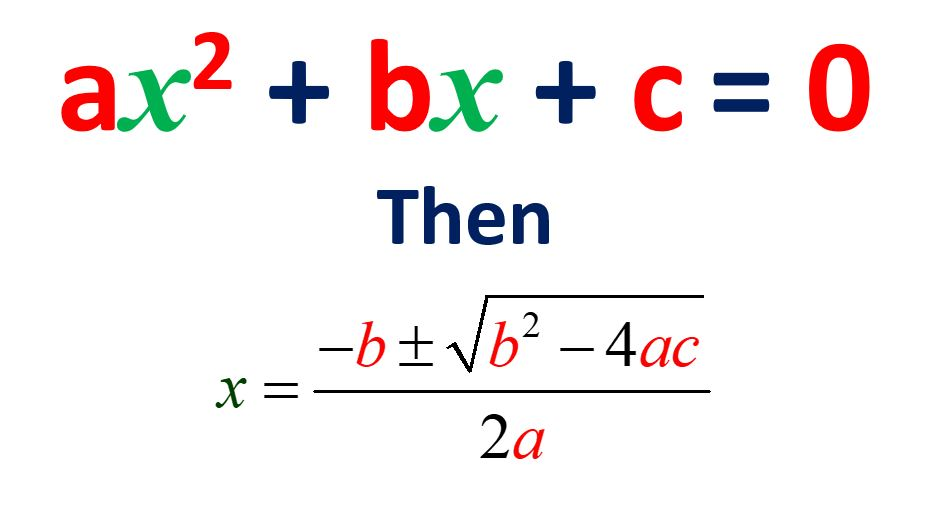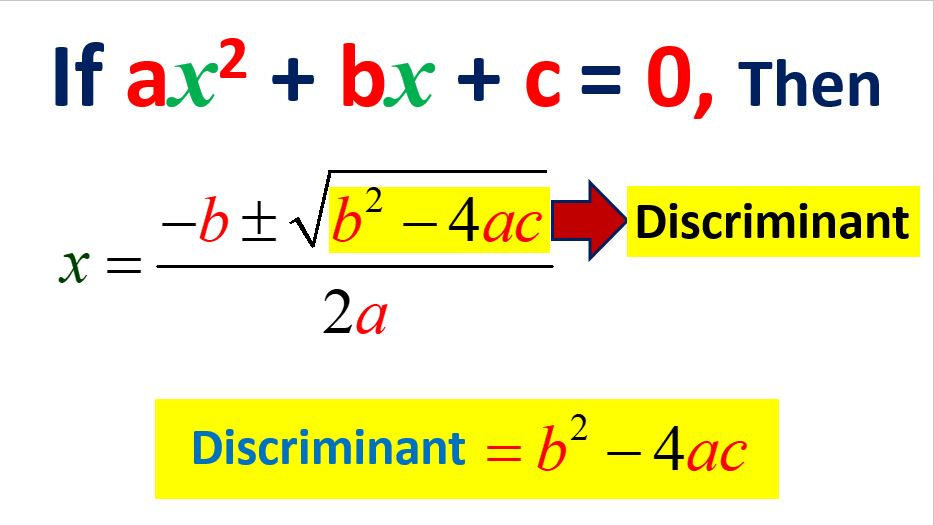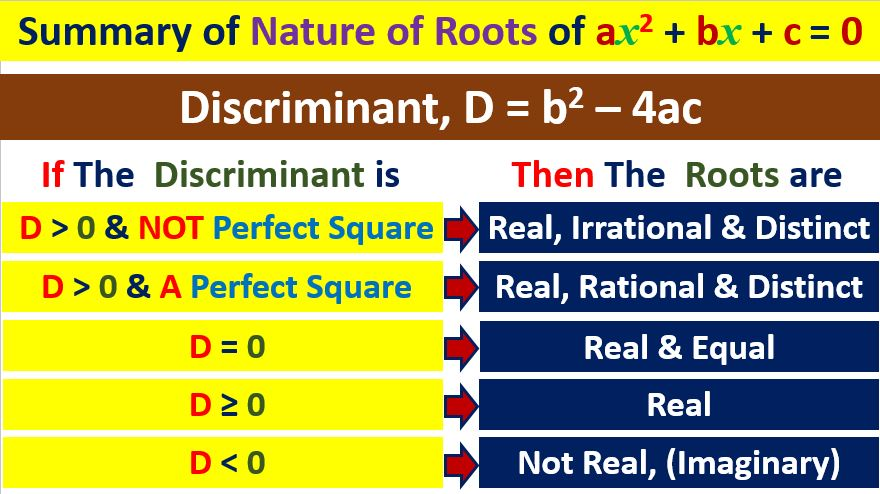Monday, September 27, 2021
Home > ICSE Solutions for Class 10 Maths > Quadratic Equations Class 10 ICSE Maths Revision Notes Chapter 5 PDF

# Quadratic Equations Class 10 ICSE Maths Revision Notes Chapter 5 PDFHi students, Welcome to Amans Maths Blogs (AMB). In this article, you will get Quadratic Equations Class 10 Maths Revision Notes Chapter 5 PDF.

A polynomial of the form of p(x) = ax2 + bx + c is known as quadratic polynomial, where a, b, c are real and a ≠ 0.

For example: p(x) = 3x2 + 5x – 2, here a = 3, b = 5 and c = -2.

An equation in the form of ax2 + bx + c = 0 is known as quadratic equation, where a, b, c are real and a ≠ 0.

For example: 3x2 + 5x – 2 = 0, here a = 3, b = 5 and c = -2.

## Solving Quadratic Equations by Factorization

To solve a quadratic equation by factorization, we do the following steps:

Step 1 : Express the equation in the form of ax2 + bx + c = 0.

Step 2 : Factorize ax2 + bx + c using middle term splitting method.

Step 3 : Put each factor equal to zero.

Step 4 : Solve each equation.

## Equations Reducible to Quadratic Equations

An equation in the form of ax2 + bx + c = 0 is known as quadratic equation, where a, b, c are real and a ≠ 0.

In some questions, the given equations are not in form of ax2 + bx + c = 0. Then, to solve such type of equations, first we need to reduce the given equation in form of quadratic equation ax2 + bx + c = 0 by using suitable algebraic transformation and then solve it.

An equation in the form of ax2 + bx + c = 0 is known as quadratic equation, where a, b, c are real and a ≠ 0.

To solve quadratic equations, there are two methods :

In this exercise, the quadratic equations are solved by using the quadratic formula.Let the quadratic equation is ax2 + bx + c = 0, where a ≠ 0. We need to find the value of x.

ax2 + bx + c = 0

ax2 + bx = – c [Transposing constant term c to RHS]

x2 + (b/a)x = – c/a [Dividing both sides by a to make coefficient of x2 = 1]

x2 + (b/a)x + b2/4a2 = b2/4a2 – c/a [Adding both sides by b2/4a2]

x2 + 2(b/2a)x + b2/4a2 = (b2 – 4ac)/4a2

⇒ (x + b/2a)2 = (b2 – 4ac)/4a2

⇒ (x + b/2a) = ± √(b2 – 4ac)/2a

x = – b/2a ± √(b2 – 4ac)/2a

x = – b ± √(b2 – 4ac)]/2a.

Therefore, if the quadratic equation ax2 + bx + c = 0, then the value of x is x = [- b ± √(b2 – 4ac)]/2a. This is known as Quadratic Formula.

Hence, there are two values of x as x = [- b + √(b2 – 4ac)]/2a or x = [- b + √(b2 – 4ac)]/2a.

An equation in the form of ax2 + bx + c = 0 is known as quadratic equation, where a, b, c are real and a ≠ 0.

Then the value of x is x = [- b ± √(b2 – 4ac)]/2a. This is known as Quadratic Formula.

Hence, there are two values of x as x = [- b + √(b2 – 4ac)]/2a or x = [- b + √(b2 – 4ac)]/2a.

Now, in x = [- b ± √(b2 – 4ac)]/2a, the term under square root, that is (b2 – 4ac) is known as the discriminant of the quadratic equation of ax2 + bx + c = 0 and it is denoted by D.

Thus, D = b2 – 4ac.## Nature of Roots of Quadratic Equations

The nature of the roots determines the types of the roots of quadratic equation of ax2 + bx + c = 0, whether the roots are real or imaginary and if the roots are real, then it will be rational or irrational and equal or unequal.

Since D = b2 – 4ac is in quadratic formula x = [- b ± √(b2 – 4ac)]/2a, then the value of the discriminant D = b2 – 4ac determines the nature of the roots of quadratic equations.

You don’t need to solve the quadratic equation of ax2 + bx + c = 0. You just need to find the value of its discriminant D = b2 – 4ac.

## How To Determine Nature of Roots of Quadratic Equations

If the discriminant D = b2 – 4ac is positive (means, D > 0), then the roots of quadratic equation are REAL and DISTINCT.

If the discriminant D = b2 – 4ac is positive and perfect square, then the roots of quadratic equation are REAL, RATIONAL and DISTINCT

If the discriminant D = b2 – 4ac is positive and NOT a perfect square, then the roots of quadratic equation are REAL, IRRATIONAL and DISTINCT.

If the discriminant D = b2 – 4ac is zero (means, D = 0), then the roots of quadratic equation are REAL and EQUAL. And, Root will be α = β = -b/2a.

If the discriminant D = b2 – 4ac is negative (means, D < 0), then the roots of quadratic equation are  NOT REAL , it means the roots are IMAGINARY.

Summary of the nature of the roots of quadratic equation is as below.